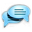Crafting and Materials - English - Ryzom Community ForumGuest# English

## Crafting and Materials

I'm writing several posts on Ryzom crafting, this one will be about available materials and their grades. Alot of information can already be found over at the Wiki (link), I try to fill some blind spots and have a more detailed look at the topic. Feel free to discuss the article in this thread, point out errors etc.

Navigation Crafting and Colors | Crafting and Materials | Crafting and Math | Online Recipe Generator | Offline Crafting Simulator

arc

# Crafting and Materials

## 0. Definitions

Average
Sum up the given numbers and divide the sum by their count.
Example: 8 + 5 + 17 = 30, now divide 30 by 3 to get an average of 10.

## 1. Theory

There are 5 material grades in Ryzom and each grade has an average value assigned to it. If you look at any material of a given grade and calculate the average value of its stats, you will end up with the following list:

• 20 for Basic
• 35 for Fine
• 50 for Choice
• 65 for Excellent
• 80 for Supreme

These values also determine the prefix of a crafted item. If you calculate the average value of the stats a crafted item displays in the preview and compare it to the chart above, you can determine the item's prefix. If your average value is above the average for the grade, the prefix will be that of the next higher grade. For example, if your average is between 51 and 65, the prefix will be Excellent. If it's at 50 it will be Choice. This seems to work for most cases, but there have been some exceptions to the rule due to rounding or other issues.

### Material Stats

All raw materials follow certain rules on how their stats are structured. There is always one value higher than all the others and two values lower than all others. The remaining values are between those extremes and equal in numbers. They represent the material average and match its grade.

The high value is 40 above the average and the two low values are 20 below the average. They cancel each other out as +40 -20 -20 is 0. The material has a perfect average value and matches the chart. So far, everything is fine. (Or excellent?)

## 2. Reality (Ingame)

If we take the above theory and apply it to an Excellent material, we end up with a high stat value of 65 + 40 = 105. For a Supreme material it is even 80 + 40 = 120!
However all materials are limited to values between 0 and 100, so those are simply cut off ingame... Is this a problem?

Yes, unfortunatelly it is. If we calculate the averages with these new values, the highs and lows don’t cancel each other out anymore. The average of the material is lower than it was supposed to be, the material is deformed.(Chart of Ryzom Material Grades)

### Example: Supreme Seed (Jewel Setting)

It has 7 stats: 1x high at 100, 2x low at 60 and the rest of its values at the grade average for supreme, which is 80. Let’s calculate the average of the material with the cut off values in mind.

(1x 100 + 2x 60 + 4x 80) divided by 7 is 77.1!

But it should have been 80 according to the theory. The Material is 3% worse than it was supposed to be! To correct this, the values of the middle stats have been changed ingame from 80 to 85.

(1x 100 + 2x 60 + 4x 85) divided by 7 is 80. Voila!(Comparison of theory and ingame stats for Supreme Sarina Seed)

General Rule
The correction amount needed for the middle stats depends on the total number of stats a material has. Let’s look at both extremes in the following paragraphs, Ammo Jacket with 4 stats and Magic Focus with 11 stats.

### Example: Ammo Jacket (Supreme Capryni Tooth)

It has only 4 attributes. If we calculate the average, we end up at:

(1x 100 + 2x 60 + 1x 80) divided by 4 is 75. Whoops.

To correct this, we have to add 20 to the middle stat, pushing it from 80 to 100! Now it looks like this:

(1x 100 + 2x 60 + 1x 100) divided by 4 is 80. Perfect!

The material appears to have two high stats now, but in reality it is just one high stat and a middle stat, which has been adjusted to make up for the wrong average.(Screenshot of a Supreme Desert Capryni Tooth information window)

### Example: Magic Focus (Supreme Beng Amber)

It has 11 attributes. If we calculate the average, we get:

(1x 100 + 2x 60 + 8x 80) divided by 11 is 78.2. Close!

This is the closest we can get towards the ideal average. It needs the smallest correction amount from 80 to 82.

(1x 100 + 2x 60 + 8x 82) divided by 11 is 79.6. If it was 83, it would be above 80.

The same principle applies to Excellent materials, the range for the middle stat in this case is 65 to 70.

Why is this important? Because once Excellent and Supreme materials are used, the ideal averages for the grades don’t match with the game anymore. The averages are an important factor for the values in the craft preview window so it is good to keep this in mind.

Thank you for reading!

---Show topic
Last visit Пт. 22 марта 2019 00:24:00 UTC
P_: